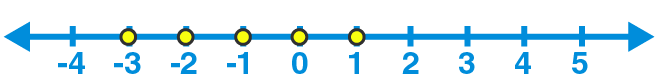If the replacement set is {-3, -2, -1, 0, 1, 2, 3}, solve the inequation $$\dfrac {(3x – 1) }{2} < 2$$. Represent its solution on the number line.

Asked by Aaryan | 9 months ago |  98

##### Solution :-

Given

Replacement set = {-3, -2, -1, 0, 1, 2, 3} and

Inequation =$$\dfrac{(3x – 1) }{2} < 2$$

3x – 1 < 2 × 2

3x – 1 < 4

3x < 4 + 1

3x < 5

We get,

x < $$\dfrac{ 5 }{ 3}$$

Therefore, solution set = {…-3, -2, -1, 0, 1}

Graphical representation of this solution set is as follows:Answered by Sakshi | 9 months ago

### Related Questions

#### Solve the inequations and graph their solutions on a number line  – 1 < (x / 2) + 1 ≤ 3, x ε l

Solve the inequations and graph their solutions on a number line  – 1 < ($$\dfrac{x}{2}$$) + 1 ≤ 3, x ε l

#### Solve the inequations and graph their solutions on a number line – 4 ≤ 4x < 14, x ε N

Solve the inequations and graph their solutions on a number line – 4 ≤ 4x < 14, x ε N

#### Solve (x / 3) + (1 / 4) < (x / 6) + (1 / 2), x ε W. Also represent its solution on the number line.

Solve ($$\dfrac{x}{3}$$) + ($$\dfrac{1}{4}$$) < ($$\dfrac{x}{6}$$) + ($$\dfrac{1}{2}$$), x ε W. Also represent its solution on the number line.

Solve the inequations ($$\dfrac{3}{2}$$) – ($$\dfrac{x}{2}$$) > – 1, x ε N